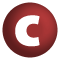# OffsetPosition

#### class OffsetPosition(source: CharSequence, offset: Int) extends Position with Product

`OffsetPosition` is a standard class for positions represented as offsets into a source document.

source

The source document

offset

The offset indicating the position

1. Product
2. Equals
3. Position
4. AnyRef
5. Any

### Value Members

1. #### def <(that: Position): Boolean

Compare this position to another, by first comparing their line numbers, and then -- if necessary -- using the columns to break a tie

Compare this position to another, by first comparing their line numbers, and then -- if necessary -- using the columns to break a tie.

2. #### def canEqual(arg0: Any): Boolean

A method that should be called from every well-designed equals method that is open to be overridden in a subclass

A method that should be called from every well-designed equals method that is open to be overridden in a subclass. See Programming in Scala, Chapter 28 for discussion and design.

3. #### def column: Int

The column number referred to by the position; column numbers start at 1

The column number referred to by the position; column numbers start at 1

4. #### def equals(arg0: Any): Boolean

The equality method defined in `AnyRef`

The equality method defined in `AnyRef`.

5. #### def hashCode(): Int

Returns a hash code value for the object

Returns a hash code value for the object.

The default hashing algorithm is platform dependent.

Note that it is allowed for two objects to have identical hash codes (`o1.hashCode.equals(o2.hashCode)`) yet not be equal (`o1.equals(o2)` returns `false`). A degenerate implementation could always return `0`. However, it is required that if two objects are equal (`o1.equals(o2)` returns `true`) that they have identical hash codes (`o1.hashCode.equals(o2.hashCode)`). Therefore, when overriding this method, be sure to verify that the behavior is consistent with the `equals` method.

6. #### def line: Int

The line number referred to by the position; line numbers start at 1

The line number referred to by the position; line numbers start at 1

7. #### def lineContents: String

The contents of the line numbered `lnum' (must not contain a new-line character)`

The contents of the line numbered ```lnum' (must not contain a new-line character). ```

8. #### def longString: String

Returns a more visual representation of this position

Returns a more visual representation of this position. More precisely, the resulting string consists of two lines:

1. the line in the document referred to by this position
2. a caret indicating the column

Example:

```    List(this, is, a, line, from, the, document)
```

definition classes: Position

10. #### def productArity: Int

return k for a product `A(x_1,`

``` return k for a product A(x_1,...,x_k) ```
``` ```
11. ``` def productElement(arg0: Int): Any for a product A(x_1, for a product A(x_1,...,x_k), returns x_(n+1) for 0 <= n < k ```
12. ``` def productElements: Iterator[Any] ```
13. ``` def productIterator: Iterator[Any] An iterator that returns all fields of this product An iterator that returns all fields of this product definition classes: Product ```
14. ``` def productPrefix: String By default the empty string By default the empty string. Implementations may override this method in order to prepend a string prefix to the result of the toString methods. ```
15. ``` val source: CharSequence ```
16. ``` def toString(): String Returns a string representation of the Position', of the form line Returns a string representation of the Position', of the form line.column' ```
``` ```
``` Instance constructors new OffsetPosition(source: CharSequence, offset: Int) new OffsetPosition() ```
``` ```